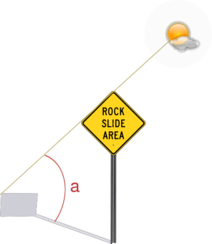homechevron_rightStudychevron_rightAstronomy

# Shadow length

Gives a vertical object shadow length for given coordinates and time. Also calculates shadow growth during whole day.Sun altitude and shadow

This simple online calculator gives a vertical object shadow length for a specified day and geographic coordinate. The calculator uses Sun position algorithm to calculate sun altitude.
Then it uses this formula to calculate shadow length:

,

where

h - object height,
a - the angle between Sun and horizon.

The calculator gives zero results if there is no shadow. As you know, this may happen at night time :).#### Shadow length

:
°
°
Digits after the decimal point: 2
Shadow length

You may also calculate the shadow growth during the whole day using the calculator below:#### Daily shadow growth

:
:
°
°
Digits after the decimal point: 2
Shadow length
The file is very large. Browser slowdown may occur during loading and creation.

URL copied to clipboard